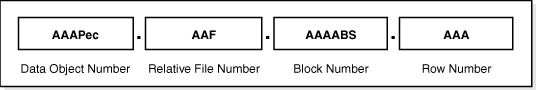## Tuesday, August 19, 2014

### Zone Map Zone ID's

Just a quick follow up to my previous post on how Zone ID's are calculated.

Let's take the following example:
```SQL> select rid, sys_op_zone_id(rid) zone_id
2   from
3   (
4    select chartorowid('AAAS5KAAHAAABYDAAA') rid
5     from dual
6   );

RID                     ZONE_ID
------------------ ------------
AAAS5KAAHAAABYDAAA 324580438021```

Recalling that extended ROWID has the following format (a nice picture from Oracle Documentation):In the binary format that would correspond to:
• Data Object Number -- 32 bits
• Relative File Number -- 10 bits
• Block Number -- 22 bits
• Row Number -- 16 bits
We know that Row Number is irrelevant for the Zone Maps because they deal with block ranges. With that in mind a simple conversion to a (base 10) number would be:

(Block Number) + (Relative File Number) * 2 ^ 22 + (Data Object Number) * 2 ^ 32

Applying the above formula to our ROWID:
```SQL> select
2    dbms_rowid.rowid_block_number(rid) +
3    dbms_rowid.rowid_relative_fno(rid) * power(2,22) +
4    dbms_rowid.rowid_object(rid) * power(2,32) base_10
5   from
6   (
7    select chartorowid('AAAS5KAAHAAABYDAAA') rid
8     from dual
9   );

BASE_10
---------------
332370368534019```

What's left after that is to slice the number into Zone Map chunk size (2^10 by default, thanks to Timur for pointing that out in the comments sections for the previous post):
```SQL> select
2    trunc((
3    dbms_rowid.rowid_block_number(rid) +
4    dbms_rowid.rowid_relative_fno(rid) * power(2,22) +
5    dbms_rowid.rowid_object(rid) * power(2,32)
6    )/power(2,10)) zone_id,
7    sys_op_zone_id(rid) sys_zone_id
8   from
9   (
10    select chartorowid('AAAS5KAAHAAABYDAAA') rid
11     from dual
12   );

ZONE_ID  SYS_ZONE_ID
------------ ------------
324580438021 324580438021```

That's all there is to it!